Next: Invariants of Modular Abelian Up: Level Raising and Lowering Previous: Level Raising and Lowering   Contents

# Problems

Let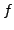be a newform in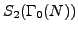(or, more generally, in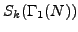). Let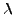be a prime ideal in the ring generated by the Fourier coefficients of. Let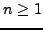be a positive integer.

Problem 7.1.1   Let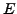be the elliptic curve 11a given by the equation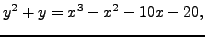and let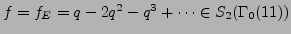be the corresponding newform. For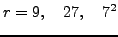compute all newforms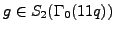with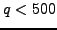prime and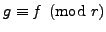. Is there a pattern?

Problem 7.1.2   Formulate a level raising conjecture modulo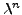. Provide computational and theoretical evidence.

Problem 7.1.3   Formulate a level lowering conjecture modulo. Provide computational and theoretical evidence.

William Stein 2006-10-20